International Journal of Business and Economics Research
Volume 5, Issue 6, December 2016, Pages: 202-209

Impact of Some Selected Macroeconomic Variables (Money Supply and Deposit Interest Rate) on Share Prices: A Study of Dhaka Stock Exchange (DSE)

Md. Nazmul Haque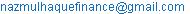Md. Nazmul Haque. Impact of Some Selected Macroeconomic Variables (Money Supply and Deposit Interest Rate) on Share Prices: A Study of Dhaka Stock Exchange (DSE). International Journal of Business and Economics Research. Vol. 5, No. 6, 2016, pp. 202-209. doi: 10.11648/j.ijber.20160506.13

Received: July 21, 2016; Accepted: July 30, 2016; Published: November 23, 2016

Abstract: This paper tries to investigate the long-run relationship and the short-run dynamics between Bangladesh stock market index (DSE General Index) and some selected macroeconomic variables such as Broad money supply (M2) and Deposit Interest rate (DIR). This study analyses monthly data for the above variables between the periods spanning from January 2009 to June 2015 and employ different econometric tools. The Johansen multivariate co-integration tests indicate that the Bangladesh stock market index (DSE General Index) and chosen two macroeconomic variables are co-integrated, this is indicative of a long-run relationship. It shows the speed of adjustment of any disequilibrium towards long run equilibrium state. The speed of adjustment is 10.7816 percent. Cointegration analysis, along with the VECM, suggests that deposit interest rate is negatively related to stock prices, and money supply (M2) is positively related to stock prices in the long-run. This study also finds short-run causal relationship. It is found that only Deposit Interest Rate has short run influence on DSE General Index. R-squared of the VECM is 0.291900 that is 29.1900 percent. It means that all of the independent variables can explain 29.1900 percent of the total variations of the dependent variable. The residuals of the regression model have serial correlation, but they are homoscedastic and normally distributed.

Keywords: Macroeconomic Variables, DSE General Index, DIR, M2, Cointegration, VECM

1. Introduction

A number of factors ranging from economic, political and Socio-cultural behavior of any country have a great impact on the stock market of that country. A number of factors occurring within or without the economic system also have impact on the stock prices of the Dhaka Stock Exchange (DSE) and it reacts either positively or negatively. Individual and institutional investors get trading facilities to exchange their holdings such as stocks, bonds and debt securities of listed companies. Investors can use some factors as a signal to expect a higher or lower return when investing in stock and one of these factors are macroeconomic variables.

The stock market of Bangladesh remained highly volatile during the last two decades. Two intense financial crises were observed during that period. First, stock markets were crashed in 1996. Second collapse was observed in the first quarter of the year 2011.

Therefore it is needed to analyze the impact of macroeconomic variables such as money supply, and deposit interest rate on the movement of DSE general index (DGEN). In this research two macroeconomic variables: Money Supply (M2), Deposit Interest Rate (DIR) and on the other hand DSE General Index are considered to understand whether the movement of stock prices of the Dhaka Stock Exchange (DSE) is subject to some macroeconomic variables change. Deposit interest rate is assumed to have negative relationship with share price and Broad money supply (M2) has positive influence over share prices.

The Dhaka Stock Exchange Limited introduced DSE Broad Index ("DSEX") and DSE 30 Index ("DS30") as per ‘DSE Bangladesh Index Methodology’ effective from January 28, 2013. But in this research, DSE General Index was used as a dummy variable.

2. Literature Review

The literature of the effects of macroeconomic variables on stock returns dates back to the late 1970s. Studies were focused on developed, emerging and both developed and emerging capital markets context and the extant literature reveals strong relationships between the macroeconomic variables and stock returns.

" examined the relationship between monthly stock returns and inflation in the post-war period from 1953 to 1974 using US data, and found a negative relationship between stock returns, in both expected and unexpected inflation." " defined the effectiveness of common stocks as an inflation hedge to the extent of which they can be used to reduce the risk of an investor’s real return which stems from uncertainty about the future level of the price of consumption goods."

", ,  and  empirically find that stock returns are negatively affected by both expected and unexpected inflation." "Based on the money demand and the quantity theory of money, , and  explain the negative linkage among stock returns and inflation." "Using post-war data for the US, Canada, Germany and the UK,  explains the relationship between stock returns and unanticipated changes in expected inflation under alternative monetary policy regimes. He finds that in countries where there is no change in the policy regime, there exists a negative relation between stock returns and changes in expected inflation." " finds that negative effect of inflation on stock return is generated by real economic fluctuations, by monetary fluctuations or changes in both real and monetary variables." " finds a moderately positive relationship between expected stock returns and expected inflation for the US and a strong positive relationship for Denmark." "The negative relationship between inflation and stock returns is supported by , , , , and ."

"Using cointegration techniques,  explains the lack of efficiency in the emerging stock markets by investigating the issue of informational efficiency in the Dhaka Stock Exchange in Bangladesh. He argued that in an efficient market the prices of the securities fully reflect all available information i.e. stock market participants incorporate the information contained in money supply changes into stock prices. Initially he tested the bivariate relationship models between stock prices and money supply changes. Results from bivariate models suggest independence between the stock price and monetary aggregates. In other words Dhaka stock market is informationally inefficient. However, it is well known that bivariate models fail to address the obvious possibility that the relationship may be driven by another variable acting on both stock price and money supply. Hence multivariate models were estimated which shows the presence of a unidirectional causality from the money (both narrow and broad) to stock price. But the findings are insensitive to the functional form of the variables employed. Thus the stock prices do not immediately reflect changes in monetary policy and the market in inefficient. One important limitation of this study is that the cointegration test conducted only for bivariate model."

" examines the causal relation between DSE stock index and a couple of macroeconomic variables like consumption expenditure, investment expenditures, real economic activity measured by GDP and industrial production index. The author employed Granger (1988) causality test and found a causal relation from stock price to consumption expenditures. He also found a unidirectional causality from investment to stock prices; weak relationship between stock price and GDP and no causal relation between stock price and industrial production index. Finally he concluded in that study that stock market is not informationally efficient in Bangladesh."

" examines the long run equilibrium and short tern dynamics between DSE stock index and a set of macroeconomic variables. In the macroeconomic variables they use money supply, 91 day T-bill rate, interest rate GDP and Industrial production index. They applied Johansen and Juselius (1990) maximum likelihood Cointegration test, Vector Error Correction Model (VECM) and also employed Granger Causality test. In the cointegration test, they found two cointegrating vectors but between them one is statistically significant. In the VECM test, they found that the lagged stock index was adjusted to long run equilibrium by percent by 43.82 percent by the combined lagged influence of all the selected macroeconomic variables. Granger causality test provides a unidirectional causality from interest rate change to stock market return."

" have attempted to trace the relationship between interest rates and rates of inflation in the economy of Bangladesh. In view of this, a time series approach was considered to examine the empirical evidence of Fisher’s effect in the country. By applying OLS and Unit Root test, the estimated value is used to determine the casual relationship between interest rates and inflation for the monthly sample period of August 1996 to December 2003. The empirical results suggest that there exist no co-movement of inflation with interest rates and the relationship between the variables is also not significant for Bangladesh. Further, the trends advocate that the inflation premium, equal to expected inflation that investors add to real-risk free rate of return, is ineffective in the country."

" have studied on the dynamic effects of deposit interest rate and Taka-US Dollar exchange rate changes on Dhaka Stock Exchange (DSE) return a well-known cointegration methodology has been applied using monthly data from January 1983 through December 2006. A change in deposit interest rate was found to have positive relation with stock price and exchange rate was found to have negative relation with stock price. A long run equilibrium and causal relationship was found between stock return and interest rate and exchange rate. However, they did not found any short run effects between stock return and two other independent variables."

" analyze long term relationship between BSE and macroeconomic variables in India. The study revealed that exchange rate and gold prices highly affected the stock prices. On the other hand the influence of foreign exchange reserves and inflation on the stock prices is up to limited extend only."

" investigates the impacts of macroeconomic variables on share price of Nigeria. He used average share price of the Nigerian Stock Exchange as dependent variables and External Debt, Inflation rate, Fiscal Deficit, Exchange rate, Foreign Capital Inflow, Investment, Industrial output as independent variables. The findings of Granger Causality test indicated that Average Share Price (ASP) does not Granger cause any of the nine (9) macroeconomic variables in Nigeria in the sample period. Only exchange rate granger causes average share price when considered in pairs. The Johansen co-integration test and showed a long run relationship between share price and the macroeconomic variables. Error correction method also showed a weak relationship between share price and macroeconomic variables. That means stock price is not a leading indicator of macroeconomic variables in Nigeria and R-square value indicated that about 60 percent of the variation in stock prices in accounted for by macroeconomic variables in Nigeria."

" investigates the impact of changes in selected microeconomic and macroeconomic variables on stock returns at Dhaka Stock Exchange (DSE). A Multivariate Regression Model computed on Standard OLS Formula has been used to estimate the relationship. Regression coefficient reveals that inflation and foreign remittance have negative influence and industrial production index; market P/Es and monthly percent average growth in market capitalization have positive influence on stock returns. All the independent variables can jointly explain 44.48 percent variation in DSE all share price index. No unidirectional Granger Causality is found between stock prices and all the predictor variables except one unidirectional causal relation from stock price and market P/Es. Finally, lack of Granger causality between stock price and selected micro and macro variables ultimately reveals the evidence of informationally inefficient market."

" examined the relationship between macroeconomic variables and stock prices in Bangladesh. Monthly data series has been used between July 2003 and October 2011 to test the relationship between DSE general index and four macroeconomic variables viz. exchange rate, M1, M2 and inflation. They have found long run equilibrium relationship between stock price and M1, M2 and inflation rate. Bivariate causality has been found from M1, M2 to stock price and from stock price to inflation."

3. Objectives of the Study

a) To measure the impact of Deposit Interest Rate on DSE General Index.

b) To measure the impact of Money Supply (M2) on DSE General Index.

4. Hypotheses

DSE General Index and Deposit Interest rate

H0: There is a correlation between DSE General Index and Deposit Interest rate.

H1: There is no correlation between DSE General Index and Deposit Interest rate.

DSE General Index and Money Supply (M2)

H0: There is a correlation between DSE General Index and Money Supply (M2).

H1: There is no correlation between DSE General Index and Money Supply (M2).

5. Research Methodology

5.1. Model Specification and Data

To investigate the impact of selected macroeconomic variables on DSE General Index (DGEN), the multiple regression technique has been applied. The regression model for predicting the DSE General Index (DGEN) is:

Y = α+ β1 X1 + β2X2+ u

Where,

Y = DSE General Index (DGEN)

α = Intercept of Y which is constant

β1 and β2 = Beta coefficients of X1 and X2 respectively

X1 = deposit interest rate (DIR)

X2 = money supply (M2)

u= error term

Monthly data are obtained from official website of Bangladesh bank and the period covered is from January 2009 to June 2015 . Data has been analyzed by computer generated results using Eviews (computer software for statistics). These time series data are used in the log form.

5.2. Unit Root Test

In time series studies, when a simple linear regression model is used to analyze the relationship among non-stationary variables, the resulting estimated equation may be a spurious one. It means that many economic time-series are integrated or nearly so. If such data are used in a regression model, it may have a very high R2 even though these variables are independent of each other. So it is important that non-stationary tests on variables should be carried out before proceeding to estimating the model. A non-stationary time series data becomes stationary series if it is differenced appropriately. If the time series data becomes stationary after it is differenced by d times, it is integrated of order d (has d unit roots). One of the common methods to find the order of integration of time series data is the unit root test. There are numerous unit root tests. One of the most popular methods is the Augmented Dickey-Fuller (ADF) test .

The Dickey-Fuller "t-statistics" for the significance of ρ is based on the estimated model :

Δxt = α + βt + πxt−1 + εt

Alternatively, in the case of autocorrelation in the observed series, to estimate the augmented Dickey-Fuller model:The null hypothesis is that xt = xt−1 + εt where εt NID (0, σ2). Under the null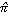will be negatively biased in a limited sample, thus only a one sided test is necessary for determining H0: π = 0 [xt I (1)] against Ha: π < 0 [xt I (0)]. The null hypothesis of unit root is rejected against the one-sided alternative if t-statistic of ρ is less than the MacKinnon critical values. This means that the variable is stationary.

5.3. Multivariate Cointegration Test and Vector Error Correction Model (VECM)

As usually macroeconomic variables are non-stationary time series, with time-dependent means and variances. But, a linear combination of non-stationary variables may be stationary. A set of variables are cointegrated, if a linear combination of the integrated series is stationary. The linear combination is called the cointegrating equation and reflects a long-run equilibrium relationship among the variables. The Johansen-Juselius procedure is used in this paper to examine for cointegration .

There are two types of Johansen test, either with trace or with eigenvalue, and the inferences might be a little bit different. The null hypothesis for the trace test is that the number of cointegration vectors is r=r*<k, vs. the alternative that r=k. Testing proceeds sequentially for r*=1, 2, etc. and the first non-rejection of the null is taken as an estimate of r. The null hypothesis for the "maximum eigenvalue" test is as for the trace test but the alternative is r=r*+1 and, again, testing proceeds sequentially for r*=1, 2, etc., with the first non-rejection used as an estimator for r.

Just like a unit root test, there can be a constant term, a trend term, both, or neither in the model. For a general VAR (p) model :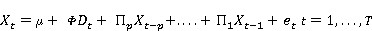There are two possible specifications for error correction: that is, two VECM (vector error correction models): VECM is a restricted VAR (vector auto regression) that has cointegration restrictions built into the specification, so that it is designed for use with non-stationary series that are known to be cointegrated. The VEC specification restricts the long-run behavior of the endogenous variables to converge to their cointegrating relationships while allowing a wide range of short-run dynamics.

1.   The long run VECM: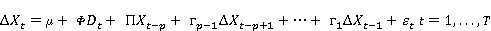Where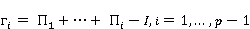2.   The transitory VECM: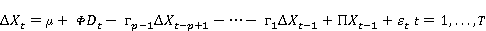Where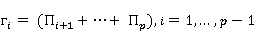Be aware that the two are the same. In both VECM (Vector Error Correction Model),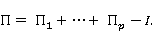Inferences are drawn on Π, and they will be the same, so is the explanatory power.

6. Analysis and Interpretation

6.1. Ordinary Least Square (OLS) Regression

In this research, it was tried to find the relationship between the dependent and independent variables through OLS regression analysis.

Table 1. Regression.

 Dependent Variable: LNDSE_INDEX Method: Least Squares Sample: 2009M01 2015M06 Included observations: 78 Variable Coefficient Std. Error t-Statistic Prob. C 7.611612 0.796834 9.552323 0.0000 LNDEPOSIT_INTEREST_RATE -0.393425 0.166281 -2.366029 0.0206 LNM2 0.201142 0.101035 1.990810 0.0500 R-squared 0.085539 Mean dependent var 8.441935 Adjusted R-squared 0.061154 S.D. dependent var 0.268113 S. E. of regression 0.259786 Akaike info criterion 0.179786 Sum squared resid 5.061658 Schwarz criterion 0.270428 Log likelihood -4.011635 Hannan-Quinn criterion. 0.216071 F-statistic 3.507777 Durbin-Watson stat 0.115317 Prob (F-statistic) 0.034969

6.1.1. T-Test

At a 5 percent level of significance, we can see that deposit interest rate and money supply (M2) are statistically significant. That is, they can significantly affect the dependent variable DSE INDEX. Here it is also seen that the relationship between the DSE INDEX and deposit interest rate is negative and between the DSE INDEX and money supply (M2) is positive, that are assumed to be so.

6.1.2. F-Test

In the above model the probability of getting F-statistic 3.507777 is 0.034969. At a 5 percent level of significance we can reject the null hypothesis of all independent variables jointly cannot affect the dependent variable. It means, all independent variables jointly affect the dependent variable.

6.1.3. R-Squared

R-squared of this model is 0.085539 that is 8.5539 percent. It means that all of the independent variables can explain 8.5539 percent of the total variations of the dependent variable. It is quite unsatisfactory result.

6.2. Augmented Dickey-Fuller Unit Root Test

Table 2. LNDSE_INDEX.

 Null Hypothesis: LNDSE_INDEX has a unit root Null Hypothesis: D (LNDSE_INDEX) has a unit root t-Statistic Prob.* t-Statistic Prob.* Augmented Dickey-Fuller test statistic -2.242070 0.1935 Augmented Dickey-Fuller test statistic -8.402699 0.0000

At 5 percent level of significance, this time series data is non-stationary at level, but stationary after first difference.

Table 3. LNDEPOSIT_INTEREST_RATE.

 Null Hypothesis: LNDEPOSIT INTEREST RATE has a unit root Null Hypothesis: D (LNDEPOSIT INTEREST RATE) has a unit root t-Statistic Prob.* t-Statistic Prob.* Augmented Dickey-Fuller test statistic -1.070236 0.7237 Augmented Dickey-Fuller test statistic -8.241117 0.0000

At 5 percent level of significance, this time series data is non-stationary at level, but stationary after first difference.

Table 4. LNM2.

 Null Hypothesis: LNM2 has a unit root Null Hypothesis: D (LNM2) has a unit root t-Statistic Prob.* t-Statistic Prob.* Augmented Dickey-Fuller test statistic -3.270508 0.0198 Augmented Dickey-Fuller test statistic -12.35578 0.0001

At 5 percent level of significance, this time series data is stationary at level and also stationary after first difference.

6.3. Johansen System Cointegration Test

Johansen proposes to test the significance of two different likelihood ratios: the trace statistics and maximum eigenvalue statistics. The trace statistics tests the null hypothesis of r cointegrating vectors against the alternative hypothesis of n cointegrating vectors. The maximum eigenvalue statistics, on the other hand, tests the null hypothesis of r cointegrating vectors against the alternative hypothesis of r +1 cointegrating vectors.

Lag length criteria (AIC: Akaike information criterion and HQ: Hannan-Quinn information criterion) suggest including 2 lag intervals. Therefore 2 lag intervals are used in Johansen System Cointegration Test.

Table 5. Cointegration.

 Sample (adjusted): 2009M04 2015M06 Included observations: 75 after adjustments Trend assumption: Linear deterministic trend Series: LNM2 LNDSE_INDEX LNDEPOSIT_INTEREST_RATE Lags interval (in first differences): 1 to 2 Unrestricted Cointegration Rank Test (Trace) Hypothesized No. of CE (s) Eigenvalue Trace Statistic 0.05 Critical Value Prob.** None * 0.256579 42.12158 29.79707 0.0012 At most 1 * 0.167619 19.88460 15.49471 0.0102 At most 2 * 0.078417 6.124705 3.841466 0.0133 Trace test indicates 3 cointegrating eqn (s) at the 0.05 level * denotes rejection of the hypothesis at the 0.05 level Unrestricted Cointegration Rank Test (Maximum Eigen value) Hypothesized No. of CE (s) Eigenvalue Max-Eigen Statistic 0.05 Critical Value Prob.** None * 0.256579 22.23698 21.13162 0.0348 At most 1 0.167619 13.75990 14.26460 0.0599 At most 2 * 0.078417 6.124705 3.841466 0.0133 Max-eigenvalue test indicates 1 cointegrating eqn (s) at the 0.05 level * denotes rejection of the hypothesis at the 0.05 level

So it is seen that these time series variables have long run association. Therefore a Vector Error Correction Model can be run.

6.4. Vector Error Correction Model (VECM)

Table 6. VECM.

 Vector Error Correction Estimates Sample (adjusted): 2009M04 2015M06 Included observations: 75 after adjustments Standard errors in () & t-statistics in [] Cointegrating Eq: CointEq1 LNDSE_INDEX (-1) 1.000000 LNM2 (-1) -0.062072 (0.16756) [-0.37044] LNDEPOSIT_INTEREST_RATE (-1) 0.267349 (0.25495) [1.04865] C -8.525515

In the above VECM, there are three models. But we have interest only on the model, where D (LNDSE INDEX) is the dependent variable and lags of D (LNDSE_INDEX), D (LNM2) and D (LNDEPOSIT INTEREST_RATE) are the independent variables. This model is known as error correction model.

Error Correction Model

Dependent Variable: D (LNDSE_INDEX)

Method: Least Squares

Table 7. Error Correction Model.

 Error Correction: Coefficient Std. Error t-Statistic Prob. CointEq1 -0.107816 0.046451 -2.321084 0.0233 D (LNDSE_INDEX (-1)) 0.085941 0.121472 0.707491 0.4817 D (LNDSE_INDEX (-2)) 0.027746 0.108691 0.255278 0.7993 D (LNM2 (-1)) 1.015965 1.072382 0.947390 0.3468 D (LNM2 (-2)) 1.358809 1.052215 1.291379 0.2010 D (LNDEPOSIT_INTEREST_ RATE (-1)) -0.573640 0.215841 -2.657690 0.0098 D (LNDEPOSIT_INTEREST_ RATE (-2)) 0.482419 0.232522 2.074721 0.0419 C -0.024904 0.025396 -0.980601 0.3303 R-squared 0.291900 Mean dependent var 0.008367 Adjusted R-squared 0.217919 S. D. dependent var 0.088442 S. E. of regression 0.078214 Akaike info criterion -2.158196 Sum squared resid 0.409869 Schwarz criterion -1.910997 Log likelihood 88.93234 Hannan-Quinn criterion -2.059492 F-statistic 3.945630 Durbin-Watson stat 2.194276 Prob (F-statistic) 0.001160

Here, -0.107816 is the coefficient of the cointegrating model. It is also called the error correction term. The cointegrating model is represented by (LNDSE_INDEX (-1) - 0.062072*LNM2 (-1) + 0.267349*LNDEPOSIT INTEREST RATE (-1) - 8.525515). In such model, there is a guideline that error correction term should be negative and statistically significant. In our model error correction term is statistically significant. As the P-value is 0.0233 which is less than 5 percent. It is also known as speed of adjustment of any disequilibrium towards long run equilibrium state. The speed of adjustment is 10.7816 percent. It is also found that in long run there is a positive relationship between the DSE INDEX and MONEY SUPPLY (M2) and a negative relationship between the DSE INDEX and DEPOSIT INTEREST RATE. The all other coefficients are known as short run coefficients, not long run coefficients. The coefficient of C is the constant term of the model.

i.      T-Test

At a 5 percent level of significance, we can see that the cointegrating equation (LNDSE_INDEX (-1) - 0.0620716780454*LNM2 (-1) + 0.267348534121*LNDEPOSIT INTEREST RATE (-1) - 8.52551460668), D (LNDEPOSIT INTEREST RATE (-1)) and D (LNDEPOSIT INTEREST RATE (-2)) are statistically significant. That is, they can significantly affect the dependent variable D (LNDSE_INDEX). All other independent variables are not statistically significant at 5 percent level of significance.

ii.    F-Test

At a 5 percent level of significance, we can reject the null hypothesis of all independent variables jointly cannot affect the dependent variable. It means, all independent variables jointly affect the dependent variable.

iii.  R-Squared

R-squared of this model is 0.291900 that is 29.1900 percent. It means that all of the independent variables can explain 29.1900 percent of the total variations of the dependent variable.

6.5. Residual Analysis

For statistical accuracy and efficiency of the residuals in the VECM, diagnostic tests are performed. A regression model is said to be more satisfactory if the residuals of the regression model have no serial correlation, and they are homoscedastic and normally distributed.

6.5.1. Breusch-Godfrey Serial Correlation Lm Test

Table 8. Breusch-Godfrey Serial Correlation Lm Test.

 F-statistic 3.35336 Prob. F (2,65) 0.0411 Obs*R-squared 7.01474 Prob. Chi-Square (2) 0.03

At a 5 percent level of significance we can reject the null hypothesis of no serial correlation. It means that there is serial correlation in the residuals.

6.5.2. Heteroscedasticy Test: Breusch -Pagan-Godfrey

Table 9. Heteroscedasticy Test: Breusch -Pagan-Godfrey.

 F-statistic 1.9726 Prob. F (9,65) 0.0569 Obs*R-squared 16.0901 Prob. Chi-Square (9) 0.065

At a 5 percent level of significance we cannot reject the null hypothesis of no heteroscedasticity. It means that the residuals are homoscedastic.

6.5.3. Normality Test: Jarque-Bera

Table 10. Normality Test: Jarque-Bera.

 Series: Residuals Sample 2009M04 2015M06 Observations 75 Mean 8.16e-18 Median -0.010224 Maximum 0.248057 Minimum -0.206587 Std. Dev. 0.074423 Skewness 0.370859 Kurtosis 3.974057 Jarque-Bera 4.684164 Probability 0.096127

At a 5 percent level of significance we cannot reject the null hypothesis of normally distribution of the residuals. It means that the residuals are normally distributed.

7. Conclusion

The study investigates the long-term relations between macroeconomic variables and the Dhaka stock market prices using Johansen’s methodology of multivariate cointegration analysis and Vector Error Correction Model. The macroeconomic forces were represented by deposit interest rates and money supply (M2) while the DSE General Index was used to represent changes in the Dhaka stock market prices. Monthly data are used from January 2009 to June 2015.

By applying ADF test, we find two variables (LNDSE_INDEX and LNDEPOSIT INTEREST RATE) are non-stationary at level and the first differences are stationary. LNM2 is stationary at level and the first difference is also stationary.

The main findings revealed that there is a long-term relationship between the stock prices and macroeconomic variables. According to the cointegration analysis and the VECM estimated in the study, the stock prices and macroeconomic variables are related significantly in the long run. The deposit interest rate was negatively related with the stock prices; this was expected as higher the interest rates, theoretically, shift investors away from stocks and vice versa. The broad money supply is positively related with the stock prices which confirm that the corporate earnings effect leads to a boost in companies’ cash flows and, hence, higher stock prices.

The results of the VECM revealed that around 10.7816% of the disequilibrium in the long-run model is corrected every month as DSE General Index reverts back to its equilibrium. However, the DSE General Index and macroeconomic variables were insignificant at most lags in the ECM specification for DSE General Index in the short run. It is found that only Deposit Interest Rate has short run influence on DSE General Index.

Diagnostic tests in the VECM were performed and it is found that there was serial correlation in the residuals but they were homoscedastic and normally distributed. In light of the analysis made in the study, policy makers and economists in Bangladesh need to be careful when they try to influence the economy through changes in key macroeconomic variables comprising the deposit interest rates and money supply (M2). Further research on the topic may also want to consider other macroeconomic variables such as call money rate, long-term government bond rate and industrial production index, which are important economic drivers and may significantly influence the stock prices.

References

1. Afzal, N. and Hossain, S.S. (2011). An empirical analysis of the relationship between macroeconomic variables and stock prices in bangladesh. Bangladesh Development Studies, Vol. XXXIV, December 2011, No. 4.
2. Ali, M. B. (2011). The impact of selected micro and macroeconomic variables on the stock prices of emerging stock market: a case of Dhaka stock exchange (DSE). International Journal of Business and Commerce Vol. 1, No. 2: October 2011, pp. 25-38.
3. Ahmed M.F. (2000). Emerging stock market and the economy. South East Asian Studies Series, Nagasaki University, Japan.
4. Ahmed. M. N. and Imam M. Osman (2007). Macroeconomic factors and bangladesh stock market. International Review of Business Research Paper, Vol. 3, No. 5, pp. 21-35.
5. Asaolu, T. O., & Ogunmuyiwa, M. S. (2011). An econometric analysis of the impact of macroeconomic variables on stock market movement in Nigeria. Asian Journal of Business Management, 3 (1), 72-78.
6. Banerjee, P.K. and Adhikary, B.K. (2009). Dynamic effects of changes in interest rates and exchange rates on the stock market return in Bangladesh. Journal of Asia Pacific Studies, 25.
7. Bodie, Zvi. (1976). Common stocks as a hedge against inflation. Journal of Finance 31 (2).
8. Chatrath, A., Ramchander, S., & Song, F. (1997). Stock prices, inflation and output: evidence from India. Applied Financial Economics, 7, 439–445.
9. Crosby, M. (2002). Stock returns and inflation. Australian Economic Papers. 40 (2): 156-165.
10. Chowdhury A.R. (1995). Is the Dhaka stock exchange informationally efficient? The Bangladesh Development Studies, vol. XXlll, pp. 89-104.
11. Dickey, D. A. and Fuller, W. A. (1979). Distribution of the estimators for autoregressive time series with a unit root. Journal of the American Statistical Association 74, pp. 427-431.
12. Engle, R. F. and Granger, C. W. J. (1987). Co-integration and error correction: representation, estimation, and testing, Econometrica55 (2), pp. 251-76.
13. Fama, E.F. (1981). Stock returns, real activity, inflation and money. American Economic Review, 71, 545-565.
14. Fama E. F. and Schwert G. W. (1977). Asset returns and inflation. Journal of Financial Economics, 5 (2): 115-46.
15. Gallagher, L. A., & Taylor, M. P. (2002). The stock return–inflation puzzle revisited. Economics Letters, 75, 147–156.
16. Geske, R. & Roll, R. (1983). The fiscal and monetary linkage between stock returns and inflation. Journal of Finance, 38, 1-33.
17. Kaul G. (1990). Monetary regimes and the relation between stock returns and inflation expectation. Journal of Financial and Quantitative Analysis, 25, (3), 307-321.
18. Marshall, D.A. (1992). Inflation and asset returns in a monetary Economy. The Journal of Finance, 47: 1315-1342.
19. Najand, M., & Noronha, G. (1998). Causal relations among stock returns, inflation, real activity and interest rates: Evidence from Japan. Global Finance Journal, 1, 71–80.
20. Nelson C. R. (1976). Inflation and rates of return on common stocks. Journal of Finance, 31: 471-483.
21. Omran, M., & Pointon, J. (2001). Does the inflation rate affect the performance of the stock market? The case of Egypt. Emerging Markets Review, 2, 263–279.
22. Sharma, G.D. and Mahendru, M. (2010). Impact of macro-economic variables on stock prices in India. Global Journal of Management and Business Research, 10 (7), 19-24.
23. Tanggaard, C. (2002). The relation between asset returns and inflation at short and long Horizons. Journal of International Financial Markets, Institutions and Money, 12, 101-118.
24. Uddin, M. G. S. and Alam, M. M. (2007). The Impacts of interest rate on stock market: empirical evidence from dhaka stock exchange. South Asian Journal of Management and Sciences, 1 (2), 123-132.
25. Zhao, X., (1999). Stock Prices, Inflation and output: evidence from China. Applied Economics Letters, 6, 509–511.
26. https://www.iei.liu.se/nek/ekonometrisk-teori-7-5-hp-730a07/labbar/1.233753/dfdistab7b.pdf
27. https://en.wikipedia.org/wiki/Johansen_test
28. https://www.bb.org.bd/econdata/index.php

BiographyMd. Nazmul Haque has completed BBA and MBA from Rajshahi University and is in teaching profession as a Lecturer in Finance, Department of Business Administration, Atish Dipankar University of Science and Technology, Dhaka, Bangladesh.
 Contents 1. 2. 3. 4. 5. 5.1. 5.2. 5.3. 6. 6.1. 6.2. 6.3. 6.4. 6.5. 7.
Article ToolsAbstractPDF(246K)